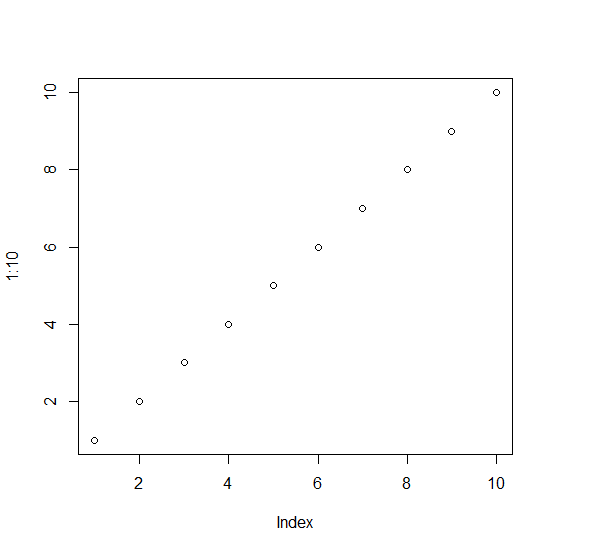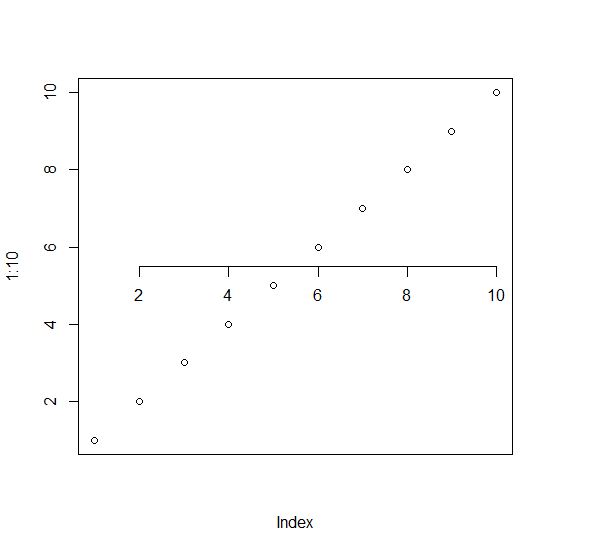# How to change the position of X-axis in base R plot?

By default, the position of X-axis is at the bottom and we know that it is always there in all software/programming tools. If we want to change the position of X-axis in base R plot then we can use axis function with pos argument. The pos argument will decide the position of the X-axis on Y-axis.

Check out the Example mentioned below to understand how it can be done.

## Example

To change the position of X-axis in base R plot, use the following command −

plot(1:10)

## Output

If you execute the above given snippet, it generates the following Output −To create a point chart with X-axis at 5.5 on Y-axis on the above created data frame, add the following code to the above snippet −

plot(1:10,xaxt="n")
axis(1,pos=5.5)


## Output

If you execute all the above given snippets as a single program, it generates the following Output −